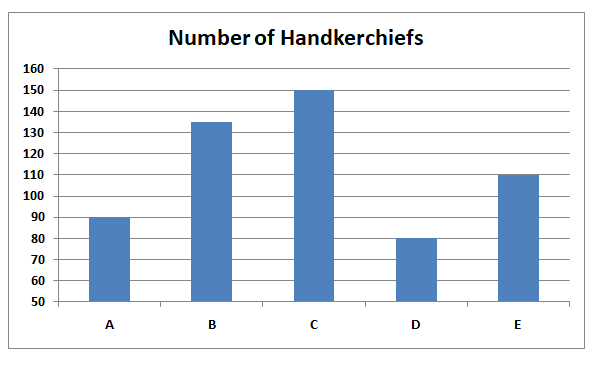# SBI Clerk Prelims 2021 Quantitative Aptitude Questions (Day-01)

Dear Aspirants, Our IBPS Guide team is providing new series of Quantitative Aptitude Questions for SBI Clerk Prelims 2020 so the aspirants can practice it on a daily basis. These questions are framed by our skilled experts after understanding your needs thoroughly. Aspirants can practice these new series questions daily to familiarize with the exact exam pattern and make your preparation effective.

Start Quiz

Directions (1-5) :Study the following information carefully and answer the questions given below.

The given bar graph shows the number of handkerchiefs sold in five different shops in 2019.1) What is the difference between the numbers of handkerchiefs sold by B and E?

A) 25

B) 30

C) 35

D) 40

E) 45

2) If total number of handkerchief manufactured in A, B and D is 120, 180 and 110, then what is the average number of unsold handkerchief in A, B and D together?

A) 30

B) 35

C) 40

D) 45

E) 50

3) The number of handkerchiefs sold in D is what percent of the number of handkerchief sold in C?

A) 51.111%

B) 52.222%

C) 53.333%

D) 54.444%

E) 55.555%

4) If the selling price per handkerchief in E is Rs.5, then find the total revenue generated by E?

A) Rs.450

B) Rs.480

C) Rs.500

D) Rs.550

E) Rs.600

5) If the number of handkerchief sold in 2020 is 10% increased by each shop, then what is the average number of handkerchief sold in all the shops in 2020?

A) 120.4

B) 124.3

C) 128.4

D) 118.6

E) 126.3

Directions (6-10): What value should come in the place of (?) in the following number series?

6) 12, 36, 90, 180, 270, ?

A) 540

B) 360

C) 320

D) 270

E) 480

7) 11, 180, 301, 350, ?, 384

A) 375

B) 380

C) 366

D) 377

E) 370

8) 9, 15, 27, ?, 69, 99

A) 36

B) 45

C) 54

D) 60

E) 63

9) 5, 7, 13, 25, 45, ?

A) 60

B) 72

C) 68

D) 78

E) 75

10) 12, 22, 17, 37, ?, 57

A) 45

B) 47

C) 37

D) 27

E) 57

Directions (1-5) :

Required = 135 – 110

= 25

Number of unsold kerchief in A = 120 – 90 = 30

Number of unsold kerchief in B = 180 – 135 = 45

Number of unsold kerchief in D = 110 – 80 = 30

Average = (30 + 45 + 30)/3 = 105/3

= 35

Required percentage = 80/150 * 100

= 53.333%

Required revenue = 110 * 5 = Rs.550

A = 90 * 110/100 = 99

B = 135 * 110/100 = 148.5

C = 150 * 110/100 = 165

D = 80 * 110/100 = 88

E = 110 * 110/100 = 121

Required average = (99 + 148.5 + 165 + 88 + 121)/5 = 621.5/5

= 124.3

Directions (6-10) :

12 * 3 = 36

36 * 2.5 = 90

90 * 2 = 180

180 * 1.5 = 270

270 * 1 = 270

11 + 132 = 180

180 + 112 = 301

301 + 72 = 350

350 + 52 = 375

375 + 32 = 384

9 + 6 = 15

15 + 12 = 27

27 + 18 = 45

45 + 24 = 69

69 + 30 = 99

5 + (1 * 2) = 7

7 + (2 * 3) = 13

13 + (3 * 4) = 25

25 + (4 * 5) = 45

45 + (5 * 6) = 75

12 + 10 = 22

22 – 5 = 17

17 + 20 = 37

37 – 10 = 27

27 + 30 = 57